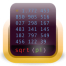A scientific calculatorCreate and insert mathematical equations into word documentsFor all quick math wizzesA great simple math program that can help you solve challenging math problemsA mathematics software used to analyze and quickly solve the problemsA software used for numerical computing and graphingSA data analysis and visualization softwareA clear cut management tool for connected devices.An interpreted development tool for engineering and scientific applicationsAn intuitive utility utilized to plot endless equation variations on a visual plot with numerical featuresA software tool for teachers and educators of geometry, algebra, statistics and calculus application!